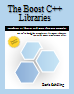The Boost C++ Libraries has been updated. The second edition was published in September 2014 and introduces 72 Boost libraries with more than 430 examples. It is available at Amazon, Barnes and Noble, for Kindle, as an Epub and as a PDF file. The second edition is based on C++11 and the Boost libraries 1.55.0 and 1.56.0 with the latter version having been released in August 2014.Find the second edition online at http://theboostcpplibraries.com/

Boost C++ 库

# 第 14 章 数据结构

## 14.2. 元组

Boost.Tuple 库提供了一个更一般的版本的 `std::pair` —— `boost::tuple` 。 不过 `std::pair` 只能储存两个值而已, `boost::tuple` 则给了我们更多的选择。

```#include <boost/tuple/tuple.hpp>
#include <boost/tuple/tuple_io.hpp>
#include <string>
#include <iostream>

int main()
{
typedef boost::tuple<std::string, std::string> person;
person p("Boris", "Schaeling");
std::cout << p << std::endl;
} ```

`boost::tuple``std::pair` 之间最重要的一点不同点： 元组可以存储无限多个值！

```#include <boost/tuple/tuple.hpp>
#include <boost/tuple/tuple_io.hpp>
#include <string>
#include <iostream>

int main()
{
typedef boost::tuple<std::string, std::string, int> person;
person p("Boris", "Schaeling", 43);
std::cout << p << std::endl;
} ```

```#include <boost/tuple/tuple.hpp>
#include <boost/tuple/tuple_io.hpp>
#include <iostream>

int main()
{
std::cout << boost::make_tuple("Boris", "Schaeling", 43) << std::endl;
} ```

```#include <boost/tuple/tuple.hpp>
#include <boost/tuple/tuple_io.hpp>
#include <string>
#include <iostream>

int main()
{
std::string s = "Boris";
std::cout << boost::make_tuple(boost::ref(s), "Schaeling", 43) << std::endl;
} ```

```#include <boost/tuple/tuple.hpp>
#include <string>
#include <iostream>

int main()
{
typedef boost::tuple<std::string, std::string, int> person;
person p = boost::make_tuple("Boris", "Schaeling", 43);
std::cout << p.get<0>() << std::endl;
std::cout << boost::get<0>(p) << std::endl;
} ```

```#include <boost/tuple/tuple.hpp>
#include <boost/tuple/tuple_io.hpp>
#include <string>
#include <iostream>

int main()
{
typedef boost::tuple<std::string, std::string, int> person;
person p = boost::make_tuple("Boris", "Schaeling", 43);
p.get<1>() = "Becker";
std::cout << p << std::endl;
} ```

`get()``boost::get()` 都会返回一个引用值。 例子中修改了 lastname 之后将会输出： `(Boris Becker 43)`

Boost.Tuple 除了重载了流操作运算符以外， 还为我们提供了比较运算符。 为了使用它们， 你必须要包含相应的头文件： `boost/tuple/tuple_comparison.hpp`

```#include <boost/tuple/tuple.hpp>
#include <boost/tuple/tuple_comparison.hpp>
#include <string>
#include <iostream>

int main()
{
typedef boost::tuple<std::string, std::string, int> person;
person p1 = boost::make_tuple("Boris", "Schaeling", 43);
person p2 = boost::make_tuple("Boris", "Becker", 43);
std::cout << (p1 != p2) << std::endl;
} ```

Boost.Tuple 还提供了一种叫做 Tier 的特殊元组。 Tier 的特殊之处在于它包含的所有元素都是引用类型的。 它可以通过构造函数 `boost::tie()` 来创建。

```#include <boost/tuple/tuple.hpp>
#include <boost/tuple/tuple_io.hpp>
#include <string>
#include <iostream>

int main()
{
typedef boost::tuple<std::string&, std::string&, int&> person;

std::string firstname = "Boris";
std::string surname = "Schaeling";
int shoesize = 43;
person p = boost::tie(firstname, surname, shoesize);
surname = "Becker";
std::cout << p << std::endl;
} ```

```#include <boost/tuple/tuple.hpp>
#include <boost/tuple/tuple_io.hpp>
#include <string>
#include <iostream>

int main()
{
typedef boost::tuple<std::string&, std::string&, int&> person;

std::string firstname = "Boris";
std::string surname = "Schaeling";
int shoesize = 43;
person p = boost::make_tuple(boost::ref(firstname), boost::ref(surname), boost::ref(shoesize));
surname = "Becker";
std::cout << p << std::endl;
} ```

`boost::tie()` 在一定程度上简化了语法， 同时， 也可以用作“拆箱”元组。 在接下来的这个例子里， 元组中的各个元素就被很方便的“拆箱”并直接赋给了其他变量。

```#include <boost/tuple/tuple.hpp>
#include <string>
#include <iostream>

boost::tuple<std::string, int> func()
{
return boost::make_tuple("Error message", 2009);
}

int main()
{
std::string errmsg;
int errcode;

boost::tie(errmsg, errcode) = func();
std::cout << errmsg << ": " << errcode << std::endl;
} ```

## 14.3. Boost.Any

Boost.Any 给我们提供了 `boost::any` 类， 让我们可以在 C++ 中像 JavaScript 一样的使用弱类型的变量。

```#include <boost/any.hpp>

int main()
{
boost::any a = 1;
a = 3.14;
a = true;
} ```

```#include <boost/any.hpp>
#include <string>

int main()
{
boost::any a = 1;
a = 3.14;
a = true;
a = std::string("Hello, world!");
} ```

```#include <boost/any.hpp>
#include <iostream>

int main()
{
boost::any a = 1;
std::cout << boost::any_cast<int>(a) << std::endl;
a = 3.14;
std::cout << boost::any_cast<double>(a) << std::endl;
a = true;
std::cout << boost::any_cast<bool>(a) << std::endl;
} ```

```#include <boost/any.hpp>
#include <iostream>

int main()
{
try
{
boost::any a = 1;
std::cout << boost::any_cast<float>(a) << std::endl;
}
{
std::cerr << e.what() << std::endl;
}
} ```

```#include <boost/any.hpp>
#include <typeinfo>
#include <iostream>

int main()
{
boost::any a = 1;
if (!a.empty())
{
const std::type_info &ti = a.type();
std::cout << ti.name() << std::endl;
}
} ```

```#include <boost/any.hpp>
#include <iostream>

int main()
{
boost::any a = 1;
int *i = boost::any_cast<int>(&a);
std::cout << *i << std::endl;
} ```

## 14.4. Boost.Variant

Boost.Variant 和 Boost.Any 之间的不同点在于 Boost.Any 可以被视为任意的类型， 而 Boost.Variant 只能被视为固定数量的类型。 让我们来看下面这个例子。

```#include <boost/variant.hpp>

int main()
{
boost::variant<double, char> v;
v = 3.14;
v = 'A';
} ```

Boost.Variant 为我们提供了一个定义在 `boost/variant.hpp` 中的类： `boost::variant` 。 既然 `boost::variant` 是一个模板， 你必须要指定至少一个参数。 Variant 所存储的数据类型就由这些参数来指定。 上面的例子就给 v 指定了 `double` 类型和 `char` 类型。 注意， 一旦你将一个 `int` 值赋给了 v， 你的代码将不会编译通过。

```#include <boost/variant.hpp>
#include <string>

int main()
{
boost::variant<double, char, std::string> v;
v = 3.14;
v = 'A';
v = "Hello, world!";
} ```

```#include <boost/variant.hpp>
#include <string>
#include <iostream>

int main()
{
boost::variant<double, char, std::string> v;
v = 3.14;
std::cout << boost::get<double>(v) << std::endl;
v = 'A';
std::cout << boost::get<char>(v) << std::endl;
v = "Hello, world!";
std::cout << boost::get<std::string>(v) << std::endl;
} ```

`boost::get()` 需要传入一个模板参数来指明你需要返回的数据类型。 若是指定了一个非法的类型， 你会遇到一个运行时而不是编译期的错误。

```#include <boost/variant.hpp>
#include <string>
#include <iostream>

int main()
{
boost::variant<double, char, std::string> v;
v = 3.14;
std::cout << v << std::endl;
v = 'A';
std::cout << v << std::endl;
v = "Hello, world!";
std::cout << v << std::endl;
} ```

```#include <boost/variant.hpp>
#include <boost/any.hpp>
#include <vector>
#include <string>
#include <iostream>

std::vector<boost::any> vector;

struct output :
public boost::static_visitor<>
{
void operator()(double &d) const
{
vector.push_back(d);
}

void operator()(char &c) const
{
vector.push_back(c);
}

void operator()(std::string &s) const
{
vector.push_back(s);
}
};

int main()
{
boost::variant<double, char, std::string> v;
v = 3.14;
boost::apply_visitor(output(), v);
v = 'A';
boost::apply_visitor(output(), v);
v = "Hello, world!";
boost::apply_visitor(output(), v);
} ```

`boost::apply_visitor()` 第一个参数需要传入一个继承自 `boost::static_visitor` 类型的对象。 这个类必须要重载 `operator()()` 运算符来处理 `boost::variant` 每个可能的类型。 相应的， 例子中的 v 就重载了三次 operator() 来处理三种可能的类型： `double``char``std::string`

`boost::apply_visitor()` 的第二个参数是一个 `boost::variant` 类型的值。

`boost::apply_visitor()` 的优点不只是“自动调用匹配的函数”这一点。 更有用的是， `boost::apply_visitor()` 会确认是否 `boost::variant` 中的每个可能值都定义了相应的函数。 如果你忘记重载了任何一个函数， 代码都不会编译通过。

```#include <boost/variant.hpp>
#include <boost/any.hpp>
#include <vector>
#include <string>
#include <iostream>

std::vector<boost::any> vector;

struct output :
public boost::static_visitor<>
{
template <typename T>
void operator()(T &t) const
{
vector.push_back(t);
}
};

int main()
{
boost::variant<double, char, std::string> v;
v = 3.14;
boost::apply_visitor(output(), v);
v = 'A';
boost::apply_visitor(output(), v);
v = "Hello, world!";
boost::apply_visitor(output(), v);
} ```

## 14.5. 练习

You can buy solutions to all exercises in this book as a ZIP file.

1. 自定义一种数据类型： `configuration` 它可以存储一个 name-value 对。 Name 为 `std::string` 类型， 而 value 可为 `std::string` 或者 `int` 或者 `float` 类型。 在 `main()` 函数里， 用 `configuration` 存储下列 name-value 对: path=C:\Windows, version=3， pi=3.1415。 通过向便准输出流输出来验证你对数据类型的设计。

2. 在输出后， 将对象中的 path 修改为 C:\Windows\System。 再次向标准输出流输出以验证你的设计。